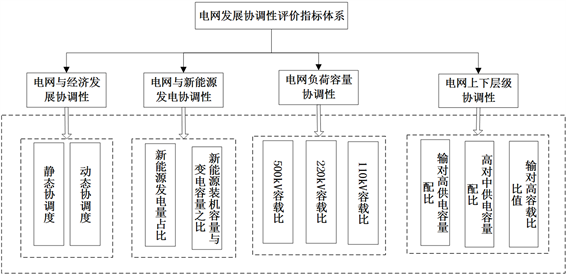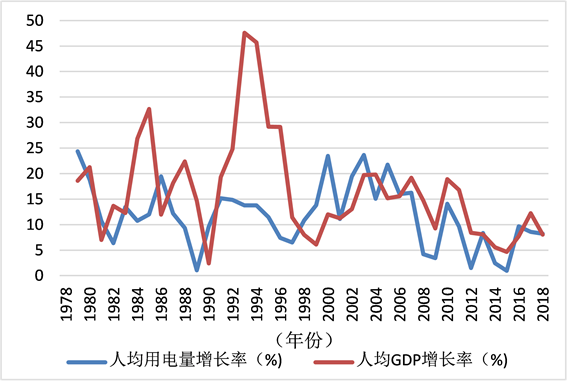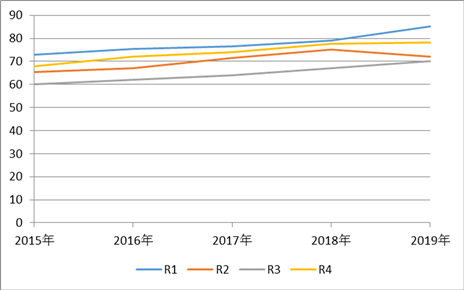# 转型发展形势下城市电网发展协调性评价Evaluation on the Coordination of Urban Power Grid Development under the Situation of Transitional Development

DOI: 10.12677/JEE.2020.83012, PDF, HTML, XML, 下载: 23  浏览: 69  科研立项经费支持

Abstract: At present, in the new stage of high-quality development of power grid construction, State Grid Corporation has proposed a strategic goal of transformational development. The new situation requires the development of urban power grids to be coordinated with the development of social economy, energy and environment. Based on relevant research results at home and abroad, this paper establishes an evaluation index system and evaluation model from the aspects of grid and economic coordination, grid and new energy generation coordination, grid load capacity coordination, and grid level coordination. Finally, a typical example is used for verification, and the evaluation results provide decision support for the high-quality and coordinated development of urban power grids in the new situation.

1. 引言

2. 电网发展协调性评价指标体系

2.1. 构建思路及指标体系Figure 1. Evaluation index system for the coordination of power grid development

2.2. 指标的计算方法

1、电网与经济发展协调性

1) 静态协调度

${D}_{1}\left(t\right)=1-\frac{|f\left(y,t\right)-\frac{f\left(y,t\right)+f\left(x,t\right)}{2}|+|f\left(x,t\right)-\frac{f\left(y,t\right)+f\left(x,t\right)}{2}|}{\frac{f\left(y,t\right)+f\left(x,t\right)}{2}}$ (式1)

${D}_{2}\left(t\right)=\frac{f\left(y,t\right)}{f\left(x,t\right)}$ (式2)

$f\left(x,t\right)$ 是电网指标对时间t发展的函数，指标主要为指标主要为：GDP，人口，固定资产投资，人均可支配收入，社会消费品零售总额及财政收入等。

2) 动态协调度

${D}_{3}\left(t\right)=\frac{1}{T}\underset{i=1}{\overset{t}{\sum }}{D}_{1}\left(t-i\right)$ (式3)

2、电网与新能源发电协调性

1) 新能源发电量占比

${\lambda }_{GQ}=\frac{\sum {Q}_{Gi}}{Q}$ (式4)

$\sum {Q}_{Gi}$ 表示i台新能源年发电量总和， $i=1,2,3,\cdots$，单位kWh；

Q表示区域供电量，单位kWh。

2) 新能源装机容量与变电容量之比

${\lambda }_{GS}=\frac{\sum {S}_{Gi}}{S}$ (式5)

$\sum {Q}_{Gi}$ 表示i台新能源年发电量总和， $i=1,2,3,\cdots$，单位kWh；

Q表示区域供电量，单位kWh。

3、电网负荷容量协调性

${R}_{si}=\frac{\sum {S}_{i}}{\sum {P}_{i}}$ (式6)

$\sum {S}_{i}$ 表示第i电压等级的总容量，单位kVA；

$\sum {P}_{i}$ 表示第i电压等级的总负荷，单位kW。

4、电网上下层级协调性

${\lambda }_{Si}=\frac{\text{}{\sum S}_{up}}{{\sum S}_{down}}×100%$ (式7)

$\text{}{\sum S}_{up}$ 表示第i电压等级的上级变电容量值，单位kVA；

${\sum S}_{down}$ 表示第i电压等级的下级变电容量值，单位kVA。

2.3. 指标的约束条件

1、电网与经济发展协调性

${D}_{1}\left(t\right)$ 由计算两变量间的相对平均离差得到，它的取值介于0到l之间。它的值越大，表明两系统间的协调性越好。

${D}_{2}\left(t\right)$ 一般介于0到1之间，也可以大于l。当其值为1时，经济及电网系统间的协调性最好，小于l或大于1分别表明电网发展滞后或超前于经济发展。

${D}_{3}\left(t\right)$ 取值介于0到l之间，其值越大，表明两系统若干年间总的动态协调度越好。

2、电网与新能源发电协调性

3、电网负荷容量协调性

500 kV及以上，[1.5, 1.8]；

220~330 kV，[1.6, 1.9]；

110 kV，[1.8, 2.0]。

4、电网上下层级协调性

220 kV变电站与110 kV变电站容量配比[0.73, 1.16]；

110 kV变电站与10 kV配变容量配比[0.72, 0.96]。

3. 电网发展协调性评价模型

3.1. 指标归一化方法

${y}_{i}=c+\frac{{x}_{i}-{m}_{i}}{{M}_{i}-{m}_{i}}d$ (式8)

${y}_{i}=\left\{\begin{array}{l}\frac{100{x}_{i}}{{a}_{i}},\text{\hspace{0.17em}}\text{\hspace{0.17em}}\text{\hspace{0.17em}}\text{\hspace{0.17em}}\text{\hspace{0.17em}}\text{\hspace{0.17em}}\text{\hspace{0.17em}}\text{\hspace{0.17em}}\text{\hspace{0.17em}}\text{\hspace{0.17em}}\text{\hspace{0.17em}}\text{\hspace{0.17em}}\text{\hspace{0.17em}}\text{\hspace{0.17em}}{x}_{i}<{a}_{i}\\ 100,\text{\hspace{0.17em}}\text{\hspace{0.17em}}\text{\hspace{0.17em}}\text{\hspace{0.17em}}\text{\hspace{0.17em}}\text{\hspace{0.17em}}\text{\hspace{0.17em}}\text{\hspace{0.17em}}\text{\hspace{0.17em}}\text{\hspace{0.17em}}\text{\hspace{0.17em}}\text{\hspace{0.17em}}\text{\hspace{0.17em}}\text{\hspace{0.17em}}{a}_{i}\le {x}_{i}\le {b}_{i}\\ 100-\frac{100{x}_{i}}{{b}_{i}},\text{\hspace{0.17em}}\text{\hspace{0.17em}}\text{\hspace{0.17em}}\text{\hspace{0.17em}}\text{\hspace{0.17em}}\text{\hspace{0.17em}}{x}_{i}\ge {b}_{i}\end{array}$ (式9)

3.2. 指标的权重计算

1、变异系数权重

$CV=\frac{\sigma }{\stackrel{¯}{x}}$ (式10)

${W}_{j}=C{V}_{j}/\underset{j=1}{\overset{m}{\sum }}C{V}_{j}$ (式11)

2、熵权法

$e=-\underset{i=1}{\overset{m}{\sum }}{p}_{i}\cdot \mathrm{ln}{p}_{i}$ (式12)

${p}_{i}=1/m\text{\hspace{0.17em}}\left(i=1,2,\cdots ,m\right)$ 时，即各种状态出现的概率相同时，熵取最大值，为： ${e}_{\mathrm{max}}=\mathrm{ln}m$

1) 计算第j个指标下第i个项目的指标值的比重 ${p}_{ij}$

${p}_{ij}={x}_{ij}/\underset{i=1}{\overset{n}{\sum }}{x}_{ij}$ (式13)

2) 计算第j个指标的熵值 ${e}_{j}$

${e}_{j}=-k\underset{i=1}{\overset{m}{\sum }}{p}_{ij}\cdot \mathrm{ln}{p}_{ij}$ (式14)

3) 计算第j个指标的熵权 ${w}_{j}$

${w}_{j}=\left(1-{e}_{j}\right)/\underset{j=1}{\overset{n}{\sum }}\left(1-{e}_{j}\right)$ (式15)

4) 确定指标的综合权数

${\beta }_{j}=\frac{{\alpha }_{i}{w}_{i}}{\underset{i=1}{\overset{m}{\sum }}{\alpha }_{i}{w}_{i}}$ (式16)

3、德尔菲法

4、组合优化权重

${W}_{i}=\frac{\underset{i=1}{\overset{m}{\sum }}{w}_{i}}{\underset{j=1}{\overset{n}{\sum }}\underset{i=1}{\overset{m}{\sum }}{w}_{i}}$ (式17)

3.3. 差异化指标修正

$A=\left[\begin{array}{cccc}{a}_{11}& {a}_{12}& \cdots & {a}_{1n}\\ {a}_{21}& {a}_{22}& \cdots & {a}_{2n}\\ ⋮& ⋮& \ddots & ⋮\\ {a}_{n1}& {a}_{n2}& \cdots & {a}_{nn}\end{array}\right]$ (式18)

${k}_{i}=\frac{{\left(\underset{j=1}{\overset{n}{\prod }}{A}_{ij}\right)}^{\frac{1}{n}}}{\underset{i=1}{\overset{n}{\sum }}{\left(\underset{j=1}{\overset{n}{\prod }}{A}_{ij}\right)}^{\frac{1}{n}}}\text{\hspace{0.17em}}\left(i,j=1,2,\cdots ,n\right)$ (式19)

3.4. 协调性综合评分

$f\left(x,t\right)=\underset{i=1}{\overset{n}{\sum }}{w}_{i}{x}_{i}$ (式20)

$f\left(y,t\right)=\underset{i=1}{\overset{n}{\sum }}{k}_{i}{y}_{i}$ (式21)

${w}_{i}$ 为权重系数；

${k}_{i}$ 为修正系数；

${x}_{i}$${y}_{i}$ 为评价指标值。

4. 实例应用

4.1. 电网与经济发展协调性分析示例Figure 2. R1 per capita electricity consumption and per capita GDP development trendTable 1. Calculation results of coordination degree between R1 power grid and economic developmentFigure 3. R1 per capita electricity consumption and per capita GDP growth rate

4.2. 指标权重综合分析结果Table 2. The weight of each evaluation index

4.3. 地区电网协调性趋势性分析Table 3. Overall coordination degree of power grids in various regions from 2015 to 2019Figure 4. Line graph of regional coordination degree in recent 5 years

5. 结语

  决胜全面建成小康社会, 夺取新时代中国特色社会主义伟大胜利[N]. 光明日报, 2017-10-28(02).  毛伟明. 统一思想, 凝聚力量, 建设具有中国特色国际领先的能源互联网企业[EB/OL]. http://www.js.sgcc.com.cn/html/main/col9/2020-04/22/20200422174834394314216_1.html, 2020-04-19.  施婧, 李玮玮, 王晓辉, 等. 基于数据包络法对配电网投入产出效益的评价[J]. 浙江电力, 2017, 36(12): 63-69.  郝伟, 朱文博. 城市电网规划项目的经济性分析指标研究[J]. 科技创新与应用, 2016(11): 193-195.  白桦. 多权重因子影响的电网规划评价指标体系[J]. 浙江电力, 2015, 34 (11): 47-51.  崔明建, 孙元章. 一种基于多层次灰色面积关联分析的电网安全综合评价模型[J]. 电网技术, 2013(12): 1-11.  高澈, 牛东晓, 马明, 等. 大规模新能源区域互联消纳能力分析及综合评价方法研究[J]. 中国电力, 2017, 50(7): 56-63.  刘新元, 宋述勇, 郑惠萍, 等. 区域电网新能源消纳多变量综合评估模型研究及应用[J]. 电器与能效管理技术, 2018(1): 39-43.  李喜兰. 考虑地区差异性电网协调发展评价方法的研究[J]. 电器与能效管理技术, 2016(18): 67-71.  王智冬, 李晖, 李隽, 等. 智能电网的评估指标体系[J]. 电网技术, 2009, 33(17): 14-18.  徐科, 刘明志, 张军, 等. 世界一流城市电网评价指标体系[J]. 电力建设, 2015, 36(11): 51-57.  雷才嘉, 贾巍, 高慧, 等. 一流城市配电网的灵活适应性评价指标及计算方法[J]. 供用电, 2019, 36(5): 2-7.  李金超. 基于GRA与PCA的电网发展协调性评估研究[J]. 电力系统保护与控制, 2010, 38(18): 49-53.  谭显东, 单葆国, 吴姗姗, 等. 经济新常态下中国全社会用电量与GDP增速差距研究[J]. 中国电力, 2017, 50(12): 5-9.  刘树森. 地市电网发展诊断指标体系研究[J]. 电气技术, 2014(5): 63-67, 76.  赵晓军, 余爽. 改革开放以来中国经济发展阶段与人力资本结构研究[J]. 经济科学, 2020, 42(1): 5-20.  杨卫红, 蔡琦, 何永秀, 等. 北京电网发展与经济发展协调性评价[J]. 华东电力, 2009, 37(10): 1627-1630.